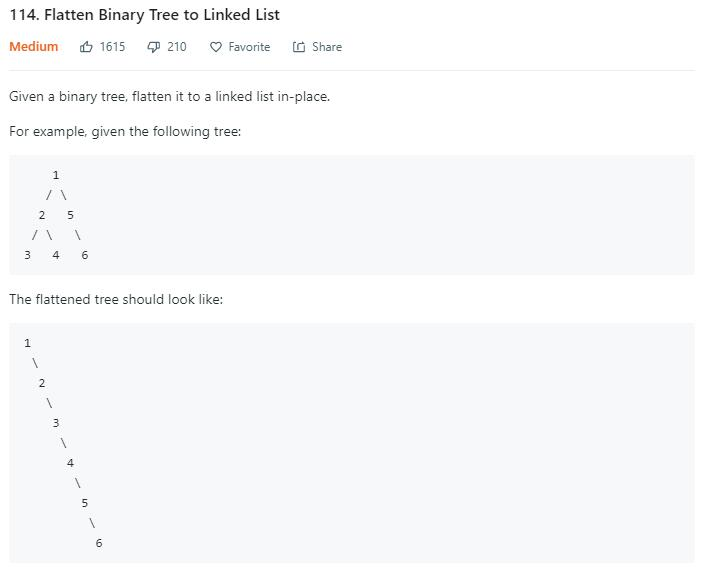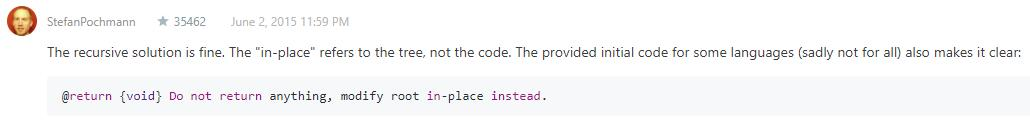# 题目描述（中等难度）# 解法一

1. 将左子树插入到右子树的地方
2. 将原来的右子树接到左子树的最右边节点
3. 考虑新的右子树的根节点，一直重复上边的过程，直到新的右子树为 null

    1
/ \
2   5
/ \   \
3   4   6

//将 1 的左子树插入到右子树的地方
1
\
2         5
/ \         \
3   4         6
//将原来的右子树接到左子树的最右边节点
1
\
2
/ \
3   4
\
5
\
6

//将 2 的左子树插入到右子树的地方
1
\
2
\
3       4
\
5
\
6

//将原来的右子树接到左子树的最右边节点
1
\
2
\
3
\
4
\
5
\
6

......


public void flatten(TreeNode root) {
while (root != null) {
//左子树为 null，直接考虑下一个节点
if (root.left == null) {
root = root.right;
} else {
// 找左子树最右边的节点
TreeNode pre = root.left;
while (pre.right != null) {
pre = pre.right;
}
//将原来的右子树接到左子树的最右边节点
pre.right = root.right;
// 将左子树插入到右子树的地方
root.right = root.left;
root.left = null;
// 考虑下一个节点
root = root.right;
}
}
}


# 解法二1
/ \
2   5
/ \   \
3   4   6


1 -> 2 -> 3 -> 4 -> 5 -> 6


... ...

... ...

    1
/ \
2   5
/ \   \
3   4   6


6 5 4 3 2 1的遍历顺序其实变形的后序遍历，遍历顺序是右子树->左子树->根节点。

public void PrintBinaryTreeBacRecur(TreeNode<T> root){
if (root == null)
return;

PrintBinaryTreeBacRecur(root.right);
PrintBinaryTreeBacRecur(root.left);
System.out.print(root.data);

}


private TreeNode pre = null;

public void flatten(TreeNode root) {
if (root == null)
return;
flatten(root.right);
flatten(root.left);
root.right = pre;
root.left = null;
pre = root;
}


public void flatten(TreeNode root) {
Stack<TreeNode> toVisit = new Stack<>();
TreeNode cur = root;
TreeNode pre = null;

while (cur != null || !toVisit.isEmpty()) {
while (cur != null) {
toVisit.push(cur); // 添加根节点
cur = cur.right; // 递归添加右节点
}
cur = toVisit.peek(); // 已经访问到最右的节点了
// 在不存在左节点或者右节点已经访问过的情况下，访问根节点
if (cur.left == null || cur.left == pre) {
toVisit.pop();
/**************修改的地方***************/
cur.right = pre;
cur.left = null;
/*************************************/
pre = cur;
cur = null;
} else {
cur = cur.left; // 左节点还没有访问过就先访问左节点
}
}
}


# 解法三

public static void preOrderStack(TreeNode root) {
if (root == null) {
return;
}
Stack<TreeNode> s = new Stack<TreeNode>();
while (root != null || !s.isEmpty()) {
while (root != null) {
System.out.println(root.val);
s.push(root);
root = root.left;
}
root = s.pop();
root = root.right;
}
}


public static void preOrderStack(TreeNode root) {
if (root == null){
return;
}
Stack<TreeNode> s = new Stack<TreeNode>();
s.push(root);
while (!s.isEmpty()) {
TreeNode temp = s.pop();
System.out.println(temp.val);
if (temp.right != null){
s.push(temp.right);
}
if (temp.left != null){
s.push(temp.left);
}
}
}


1 -> 2 -> 3 -> 4 -> 5 -> 6


... ...

public void flatten(TreeNode root) {
if (root == null){
return;
}
Stack<TreeNode> s = new Stack<TreeNode>();
s.push(root);
TreeNode pre = null;
while (!s.isEmpty()) {
TreeNode temp = s.pop();
/***********修改的地方*************/
if(pre!=null){
pre.right = temp;
pre.left = null;
}
/********************************/
if (temp.right != null){
s.push(temp.right);
}
if (temp.left != null){
s.push(temp.left);
}
/***********修改的地方*************/
pre = temp;
/********************************/
}
}